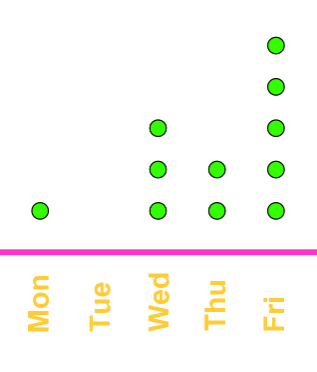# Frequency tables and dot plots

### Frequency tables and dot plots

#### Lessons

In this lesson, we will learn:

• Analyze the Frequency Table
• Understand the Dot Plot
• Convert a Frequency Table Into a Dot Plot

• Frequency table: a table showing the data values and their corresponding frequencies.
• Dot plot: a simplified version of a histogram. Each dot represents a value in that particular category.
• Dot plots are not the same as scatter plots:
• Scatter plots have a set of axes whilst dot plots do not.
• Each dot in a dot plot has a value of 1. As for a scatter plot, we need to refer to the axis for the value of a dot.
• Introduction
Introduction to Frequency Tables and Dot Plots
a)
What is a Frequency Table?

b)
What is a Dot Plot?

• 1.
Analyze the Frequency Table
Stephen is doing a questionnaire about what movie genres his classmates like and he has made the following frequency table:  Movie genre frequency Comedy 4 Action 1 Horror 7 Sci-fi 2 Thriller 6
a)
How many students like horror movies?

b)
If each student could only pick one genre and all students voted, how many students are there in the class?

c)
What is the range?

• 2.
Understand the Dot Plot
The following dot plot shows the number of student(s) who showed up to a daily meeting:a)
How many students were there on Tuesday?

b)
What is the range?

c)
How many students showed up during the first 3 days?

• 3.
Convert a Frequency Table Into a Dot Plot
The below data set shows the average number of subjects each student takes.
4  6  3  3  4  1  3  2  7  4  7  1  3
a)
Reflect the data set on a frequency table.

b)
Convert the frequency table from (a) to a dot plot.

c)
What is the range?